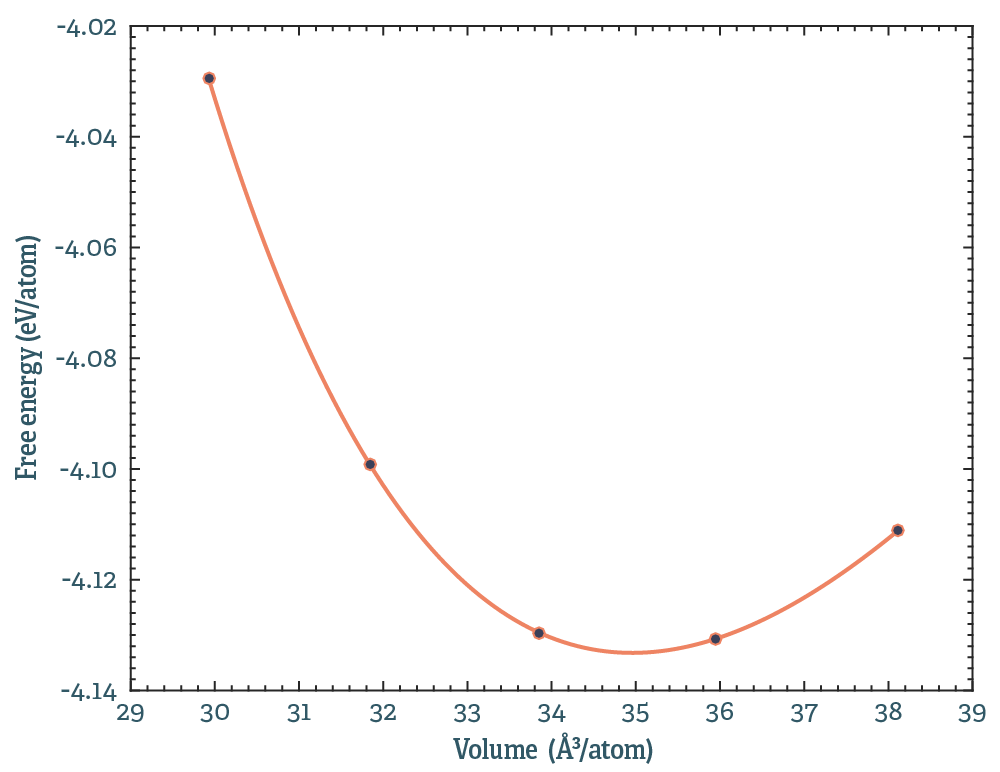### Basic free energy calculations

Navigate to /example_4_free_energy. There you should see 5 folders, each corresponding to a simulation at fix temperature, but different volumes. This particular case is PbTe at 600K. Copy all the contents to a new folder.

First, we will get the free energy at a single volume. Pick one of the folders, perhaps volume_1. We will constrain ourselves to second order force constants, run:

extract_forceconstants -rc2 100 -U0
ln -s outfile.forceconstant infile.forceconstant
phonon_dispersion_relations -loto --dos -qg 36 36 36 --temperature 300


This will produce plenty of output. The first lines calculates the second order forceconstants, including the option of calculating the renormalized baseline energy. You should have read about forceconstants already, and perhaps this is a good time to familiarize yourself with free energy calculations a bit. I put a decent explanation of the different terms here. Glance through the documentation and see what the different command line options do.

The things you need for the free energy is the renormalized baseline, $U_0$, and the phonon free energy $F_{\textrm{ph}}$. These will be in outfile.U0 and outfile.free_energy respectively. If everything worked out ok, you should have something like

Fph = -0.26006391 eV/atom
U0  = -3.76922936 eV/atom


So, by adding these numbers you get the Helmholtz free energy. Electronic entropy will be included, provided that it is in $U_0$. It is up to you to ensure that the underlying DFT calculations include that properly.

You can't get that much useful information from a single point in volume-temperature space, lets get the free energy for all the volumes, conveniently done with a small script:

#!/bin/bash
# Get the forceconstants and free energy for every volume
for i in 1 2 3 4 5
do
cd volume_${i} extract_forceconstants -rc2 100 -U0 ln -sf outfile.forceconstant infile.forceconstant phonon_dispersion_relations --dos --temperature 600 -qg 32 32 32 --loto cd .. done  This should result in a list of volumes and energies (it does not appear magically, you have to fetch the numbers):  Volume U0 Fph 29.92236913 -3.76922936 -0.26006391 31.84747692 -3.82606181 -0.27327257 33.85343312 -3.84306260 -0.28655016 35.94026710 -3.82961324 -0.30108423 38.11284394 -3.80467151 -0.30650145  Plotting this, and fitting to an equation of state (Birch-Murnaghan) should like like this:Make sure you can reproduce this. #### Small improvement First thing we can improve is how the forceconstants are extracted. In the script above, we do a full symmetry analysis for each of the volumes. That is fine, but unnecessary. The symmetry of each supercell is the same. If we modify the script slightly #!/bin/bash # Got to the first volume and do the symmetry analysis cd volume_1 extract_forceconstants -rc2 100 mv outfile.forcemap.hdf5 ../ cd .. # Reuse the symmetry analysis and get the free energy for i in 1 2 3 4 5 do cd volume_${i}
ln -sf ../outfile.forcemap.hdf5 infile.forcemap.hdf5

If you try it out, you will find that it runs a little faster. Nothing significant in this small case, but for production quality calculations this is useful. Using the same infile.forcemap.hdf5 is also a good idea, which will become apparent later.
• Use subsets of the simulation to calculate $U_0$ (using --stride, explained here), what does that convergence look like?